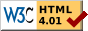#Boost C++ Libraries

...one of the most highly regarded and expertly designed C++ library projects in the world.

This is the documentation for a snapshot of the develop branch, built from commit 31c6e1f53a.

# Binders

The header functional.hpp provides enhanced versions of both the binder function object adapters from the C++ Standard Library (§20.3.6):

• binder1st
• binder2nd

As well as the corresponding helper functions

• bind1st
• bind2nd

The key benefit of these adapters over those in the Standard Library is they avoid the problem of references to references.

### Usage

Usage is identical to the standard binders. For example,

```class Foo {
public:
void bar(std::ostream &);
// ...
};
// ...
std::vector<Foo> c;
// ...
std::for_each(c.begin(), c.end(),
boost::bind2nd(boost::mem_fun_ref(&Foo::bar), std::cout));
```

### References to References

Consider the usage example above

```class Foo {
public:
void bar(std::ostream &);
// ...
};
// ...
std::for_each(c.begin(), c.end(),
boost::bind2nd(boost::mem_fun_ref(&Foo::bar), std::cout));
```

If this had been written using std::bind2nd and std::mem_fun_ref, it would be unlikely to compile.

The problem arises because bar takes a reference argument. The Standard defines std::mem_fun_ref such that it creates a function object whose second_argument_type will be std::ostream&.

The call to bind2nd creates a binder2nd which the Standard defines as follows:

```template <class Operation>
class binder2nd
: public unary_function<typename Operation::first_argument_type,
typename Operation::result_type> {
...
public:
binder2nd(const Operation& x,
const typename Operation::second_argument_type& y);
...
```

Since our operation's second_argument_type is std::ostream&, the type of y in the constructor would be std::ostream&&. Since you cannot have a reference to a reference, at this point we should get a compilation error because references to references are illegal in C++ (but see C++ Standard core language active issues list).

The binders in this library avoid this problem by using the Boost call_traits templates.

Our constructor is declared

```binder2nd(const Operation& x,
typename call_traits<
typename binary_traits<Operation>::second_argument_type
>::param_type y)
```

As a result, y has a type of std::ostream&, and our example compiles.Revised 02 December, 2006